Whiteboard Web
SDR »

# I/Q Data for Dummies

This is a description of using I/Q Data (aka "analytic signal") representing a signal. Since the topic may be quite confusing, I've described the same thing here from different point of views. If you find the information somewhat redundant, it is because it is. Different views may appeal to different readers, and if something seems unclear, keep on reading and it may be more comprehensible later - hopefully.

## Why I/Q Data?

I/Q Data is a signal representation much more precise than just using a series of samples of the momentary amplitude of the signal. Have a look at the following signal below.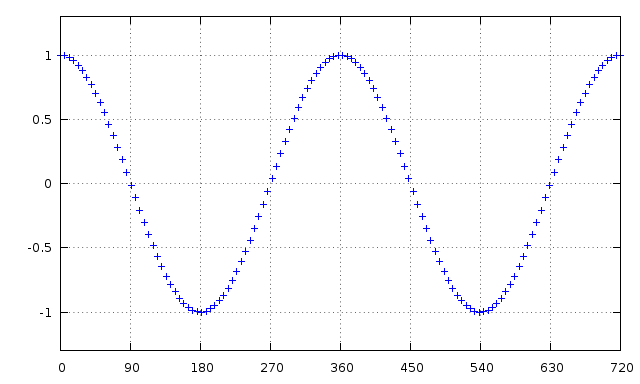This is what you may be used to work with. So why I/Q Data - isn't this good enough?

Not really. We have a few problems here.

• First, it is impossible to determine the frequency of this signal. Sure, it looks simple enough, just look at the period length? True, but you have no clue if it's a positive or negative frequency since they both generate the same curve. I.e. cos(x) = cos(-x). This becomes a problem working with the signal. Mixing (multiplying) two signals and it'll cause multiple solutions due to the uncertainty of the sign: f1 ⊗ f2 equals f1 + f2 as well as f1 - f2.
• Second, it's hard to determine the power (peak amplitude, envelope) of the signal. Basically you can only see the peak amplitude here at 0°, 180°, 360° etc, and how do you know the power is the same everywhere else as well? And did you sample the signal exactly at its peak? You really don't know.

I/Q Data solves this. Instead of looking at the signal as a flat curve as above, look at it as a corkscrew (helix, spiral, coil spring) in three dimensions.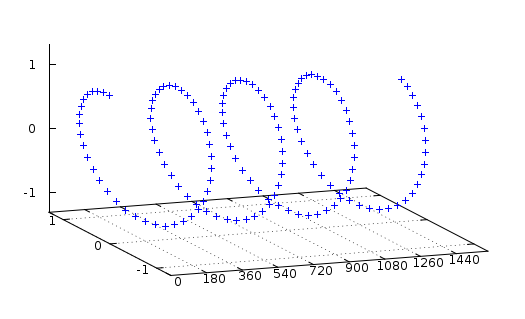Now if you look at this curve from the side, you'll actually get the same graph as the first one above. Your "real" signal actually is this 2D projection of this corkscrew signal. This is your "I" in I/Q data.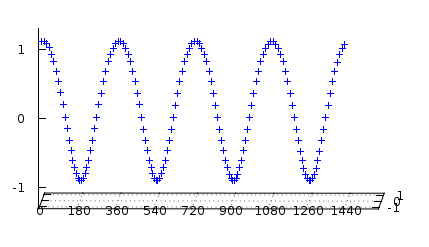Now have a look at the corkscrew from above. This looks quite similar, but as you see, it is out of phase 90° starting at zero, not at one as the other. This this the Q part of your I/Q data.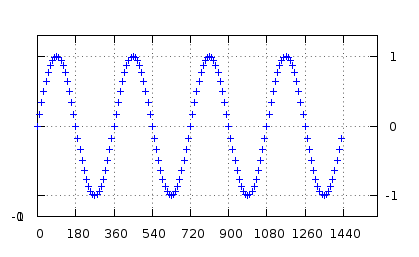Now looking at the corkscrew down the time axis you'll see it winds counter-clockwise. This means we know the frequency is positive. It could have wound clockwise as well, still generating the same I-signal (projection) but different Q-signal, representing a negative frequency.

You also see that the radius of the corkscrew is constant at every sample, if small in I large in Q and vice versa. The radius is the peak amplitude of your signal.

The axes are of course 90°, so the radius must be equal to (I²+Q²)1/2. This is the peak amplitude of your signal, and as you can see you know this for each and every sample.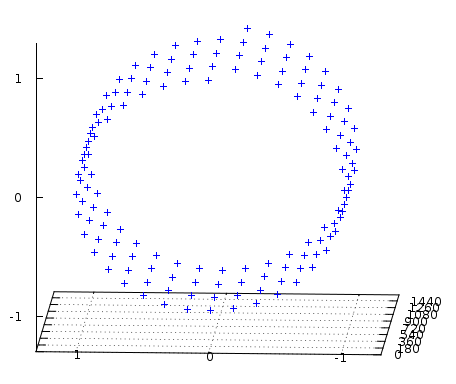## What is I/Q Data?

AS you now understand, the I/Q Data Sample is the coordinates of your signal as seen down the time axis of the corkscrew.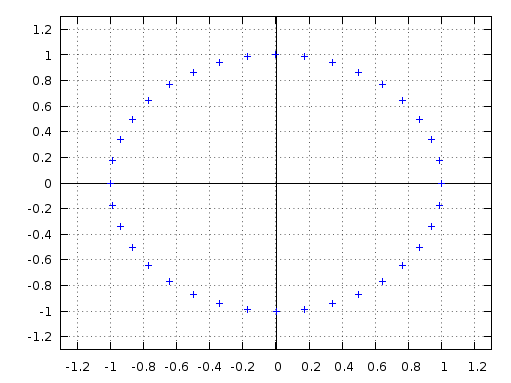You might object that your signal isn't a pure cosine function as the one we have shown here, and it might be very true. Still, every single sample of your signal can be described as such, i.e. with a peak amplitude times cosine of some phase angle.

Every single point of your signal can be described as the function A⋅cos(ϕ)

Since you may freely choose any amplitude A and angle ϕ this must of course be true (as long as the signal is continuous). The value of A⋅cos(ϕ) is the I component of the I/Q signal, i.e. your real signal. Note that this only describes your signal in one single point, i.e. one sample. Next sample gives you a new I and Q very likely resulting in another amplitude and/or phase angle, reflecting the modulation of the signal.

## One sample I/Q Data

Ok, lets take one sample of I/Q Data and see what it represents. This is also called a phase vector, or phasor.

```I = 0.69
Q = 0.40
```

Lets draw this in the complex plane.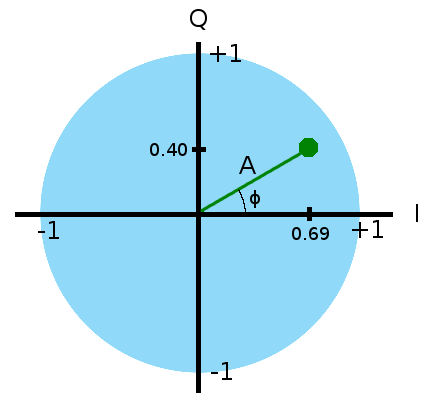Lets see what this tells us about our data point.

• The momentary amplitude of our real signal is by definition I, i.e. 0.69
• Pythagoras tells us the amplitude A of the cosine wave is `(0.69²+0.40²)1/2 = 0.8`
• Trigonometry tells us our angle is +30° into our cosine wave.

- Hold it, you say, what cosine wave?

Well, I/Q actually assumes your real signal (I, that is) can be described as the function I = A⋅cos(ϕ)

Since you are free to choose A and ϕ this must of course be true, as long the function is continuous. Remember we are looking at one single sample now, i.e. one point in time.

So by using IQ Data we not only get the momentary values of our signal, but the function generating it as well. If we put above together we get:

The real signal I = 0.8⋅cos(30°)

• I/Q Data is the representation (data type) of this cosine function.

I/Q Data is the rectangular representation of the polar notation we used above. There is a unique transformation between the two, and the different notations have different properties calculating with them. The rectangular form of I/Q Data is chosen due to the ease of hardware implementations of the most common operations.

I/Q Data consists of I and Q represented as two separate variables, a vector of length two, or more often, the complex number I + Qi (yes, I is the real part).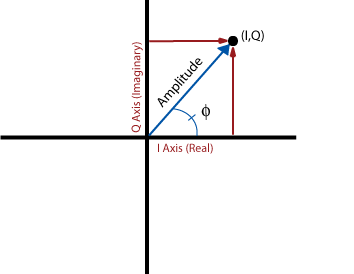Note that the Amplitude above is the waves peak amplitude, not the momentary amplitude.

• I is the current momentary amplitude of the signal (i.e. the Real signal)
• Q is the momentary amplitude of the signal phase shifted -90°.

For a simple function such as sine, the phase shift is what the signal was earlier in time, but for a signal with more than one sine component, Q reflects a -90° shift of the individual components, and not the composite signal as such. To convert a Real Signal to a I/Q Data Signal, discrete Fourier transformation is required (Hilberts transform).

## Different ways of representing the same I/Q Data Sample

There are at least three common ways to represent the I/Q Data Sample. Different representations gives you different pros and cons. Some are more easy to add, other are more easy to multiply etc. This may be important in the implementation, resulting in less complex hardware/software using the best representation.

### The rectangular form

The I/Q Data on the form Q and I is called "rectangular" (or "Cartesian") form as it can be viewed as positions in a coordinate system. I and Q are the x and y axis respectively. This is the most common representation you are used to. This form is most common due the ease of modulating/demodulating it in hardware. More about that later.

• As a complex number: I + Qi
• As a vector [I,Q]
• Or just the two plain variables I and Q

### The polar form

• Amplitude and angle

I = Amplitude⋅cos(angle)
Q = Amplitude⋅sin(angle)

The Amplitude is the peak amplitude of the cos (and sin) function, and the angle is how far into the period from zero to 360° you are (or 0 to 2π if you prefers radians).

### Eulers form

Since cos(ϕ) + i⋅sin(ϕ) = e we can write our IQ sample as

Ae

This might (not?) be the most intuitive representation of the sample. ϕ rotates the angle as seen in the polar representation, and A is of course the amplitude. Realizing this, Eulers identity becomes obvious. Because ϕ is the rotation of the vector in the complex plane, rotating it half a turn, 180° or π radians, results in a real part of -1 and no imaginary part, hence:

eπi+1 = 0

"The student should find this to be immediately obvious,
otherwise he'll never be a first rate mathematician"

-- Carl Friedrich Gauss

## Positive versus negative frequency

It is now easy to see that using I/Q we can represent the signal frequency either as positive or negative. Have a look at the two I/Q signals red and blue below to the left and compare them with their corresponding real projections. It is as obvious they differ in signs in I/Q, as it's impossible to determine the signs using only the real signal component (neither the I nor the Q projection separately).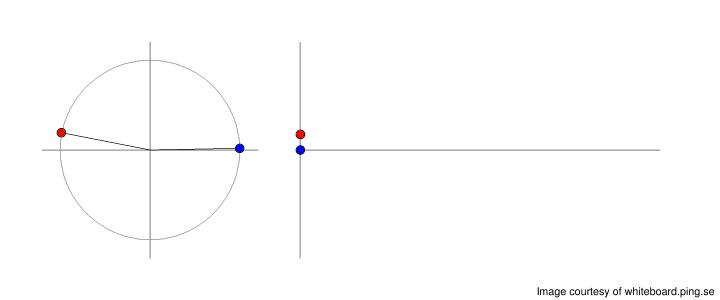(sidenote: I've put them slightly out of phase compared to each other since else they wouldn't be possible to distinguish at all in the real representation to the right. Also, please note I'm here, quiet unconventional, using the x axis in the phasor for the imaginary Q)

The same signal (well, more or less) in a 3D representation.

The I components (side view):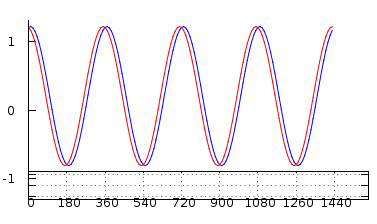The Q components (top view):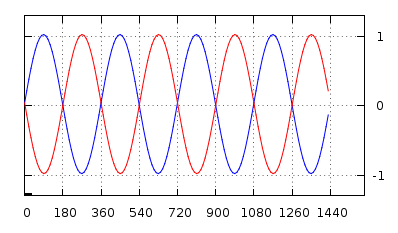The I/Q signals in 3D: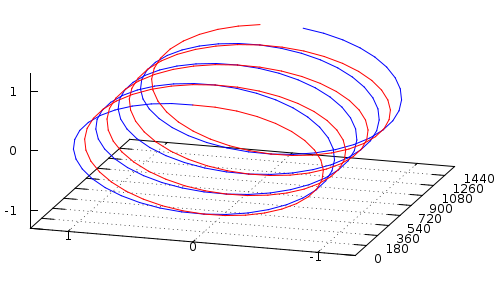As the Nyquist–Shannon sampling theorem states you can only represent frequencies up to `f/2` using a samplings rate of `f`. This is still true using IQ Data, but since you now can represent negative frequencies the signal spans `[-f/2..+f/2]` compared to `[0..+f/2]` using a ℝeal signal, hence the range is in effect doubled. Using a sampling rate of `f` and you now can represent a signal range of `f` as well. Two to the price of one!

## Mixing and multiplying signals

Using real signals or IQ Signals gives different results when you multiply them. This is because using only the real component it's not possible to uniquely determine the phase angle of the signal, hence impossible to distinguish a positive frequency from a negative.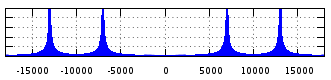Multiplying two signals f1 and f2 in the real domain:

±f1 ⊗ ±f2 = (±)f1 ± f2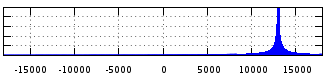Using IQ Data the signs are now given, and the result is unambiguous:

f1 ⊗ f2 = f1 + f2

A frequency spectrum in the real domain usually never show the negative side, since it always must be symmetric around zero due to the uncertainty of the sign of the frequency of the real signal -- hence the parentheses around the sign of `f1` in the first formula mixing the real signals. I've included the negative side here for illustrative purposes, despite of its redundancy.

Multiplying two complex number is easiest understood in the polar representation. The amplitude is multiplied and the angle added.

A1⋅e1⋅A2 e2 = A1A2 ei(ϕ12)

Realizing the angle is added under multiplication makes it obvious that the frequencies are added as well.

### And in time domain ...

Now let us have a look at this in time domain. To make it easier (doable!) to calculate the DFT in our heads, we choose really simple numbers. Let's mix `f` with `-f`. Using I/Q the result would be zero, not using I/Q, it would be zero and `2f` (and `-2f`, but in real it's no difference). Well, `2f`, since the zero (DC) component ... is zero, i.e. not there. I told you we'd use simple figures. :-)

Here we have `f` and `-f` as blue and red. Green is the product of the complex signals, and black is the product of the corresponding real signals.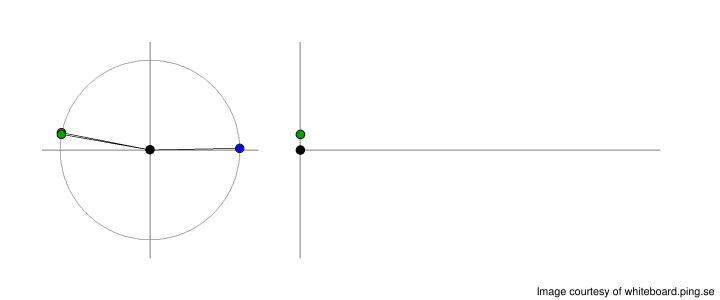Right. Green is quite right zero in frequency, and the real (ℝ) signal black is very clearly `2f` (the DC component vanishes quite naturally in the real world).

Please ponder over the fact that the green mix of the I/Q signals still has full amplitude despite of the fact that the frequency is zero. This is perfectly possible representing a DC component in I/Q like this, where the black DC component using real just vanishes. Don't worry that the green mix isn't perfectly aligned to the axis though, this is just because blue and red I/Q signals are slightly out of phase.

(and yes, I'm using the y-axis for the real signal here)

### A more complex example

If we now look at the same signals as in the frequency domain above, mixing 10 kHz (red) with 3 kHz (blue) we get the result using I/Q (green) or using real (black) as seen below.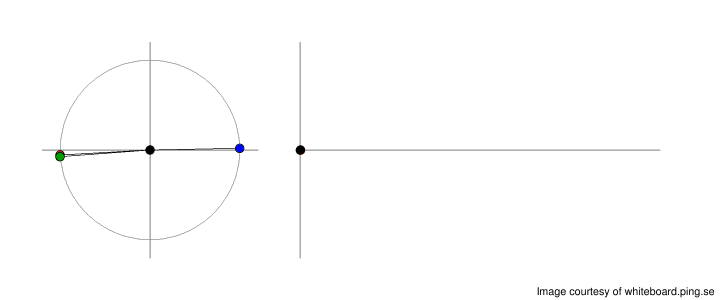Worth noticing here, is that the I/Q mix (green) is a pure sine wave of higher frequency, but the real mix (black) is clearly a composite of several frequencies (10-3, 10+3), exactly as in seen the frequency spectrum above.

## RF Down Converting to I/Q Data

There is one fundamental difference between a baseband and modulated RF signal. The modulated signal rides on a carrier of a given frequency, but the base band signal has no fixed frequency at all. Because of this, we have the possibility to encode the two-dimensional I/Q signal onto the one-dimensional RF signal without losing anything. Magic!

The carrier of a given frequency has two parameters we can change, its amplitude and phase. This is what we use to encode our I/Q data. We can encode it on carrier of frequency f like this (t for time):

Modulated Carrier RF = I⋅cos(2πft) + Q⋅sin(2πft)

By adding a cosine with its corresponding sine component of the same frequency (i.e. the carrier) we change the phase and amplitude of the resulting RF signal. Transforming it back is as easy.

```I = lowpass(RF⋅cos(2πft))
Q = lowpass(RF⋅sin(2πft))
```

Got it? The carrier has a predefined frequency, hence a fixed phase as a reference. The baseband not, hence the need of explicit representing the phase using two parameters.

Still even if this looks fine on paper, in reality the phase may drift due to the fact that the senders and receivers oscillators are never in perfect sync, but differs a little both in phase and frequency. Therefor the senders I and Q may be out of phase compared to the receivers I and Q, but the relative angle of I and Q is always correct enough, as well as the amplitude.

Negative frequencies not a problem neither. Since the carrier is of much higher frequency than the modulation, a negative signal frequency still generates a positive carrier frequency. It's first when you remove baseband from the carrier you'll need to have a way of representing it negative again, i.e. using I/Q data.

## Formulas

Some formulas calculating with I/Q Signals translating between polar and rectangular form etc.

Peak Amplitude A = (I²+Q²)½

Phase Angle ϕ = tan⁻¹(Q/I)

I = A⋅cos(ϕ)

Q = A⋅sin(ϕ)

Converting IQ Data to a plain signal: I is the original signal.

Euler form: A⋅e = A⋅(cos(ϕ) + i⋅sin(ϕ)) = I + Qi

## Some Examples

The examples below may look quite pretty, but interpret them with a grain of salt. The modulated carrier signal isn't actually represented using I/Q data. More about how to derivate the I/Q data signal from the non I/Q data modulated carrier later, once I've figured out a pedagogic explanation. :-)

### AM-modulation in IQ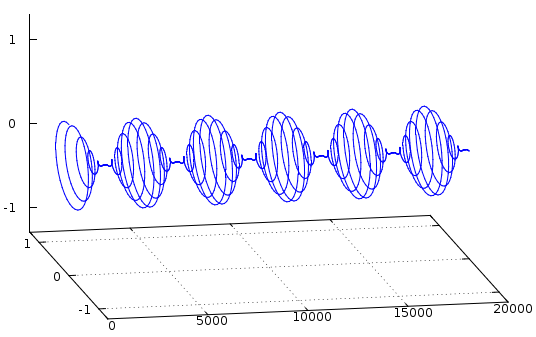Same graph seen from side, i.e. only as I.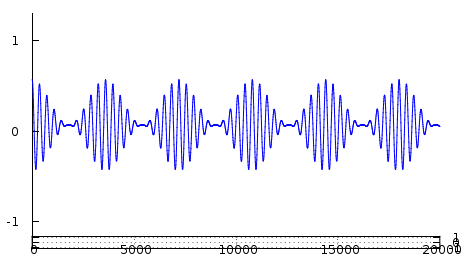The amplitude is given as (I²+Q²)½ for every sample, i.e. even for samples with the real component I equals zero.

### FM-modulation in IQ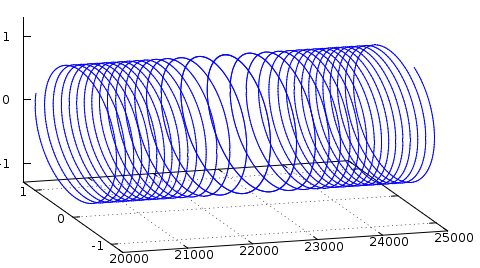Note that the amplitude is constant.

Same graph as seen from the side, i.e. as I only.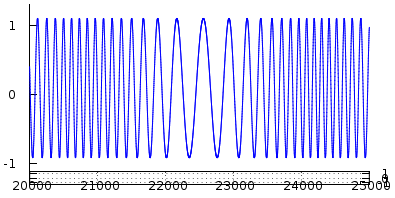### A little trickery

Have a look at the following signal in I (real) only.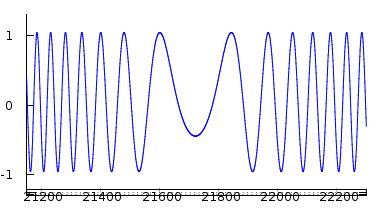Three parts, left, center and right. The frequency to the left is about the same as the frequency to the right, right? Yes, if you only got I, you can't tell the difference. But now look at the same signal in I/Q.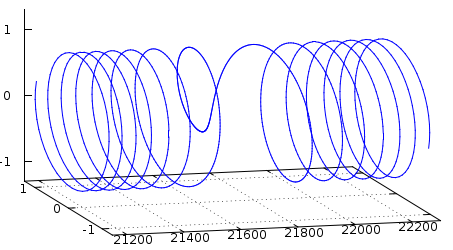You see here that the signal changes its direction at the center, i.e. goes from a positive frequency to a negative. To detect this, you need to use I/Q. The real part I alone isn't enough. This is why a signal in the real domain (I only) always is symmetric around zero in the frequency domain. This is not the case with I/Q signals.

## I/Q - just a mathematical construction?

Sometimes I get the objection: "I/Q data is nice, but it's just a mathematical construction. The true signal is real".

I do not agree with this. I'd say the true signal is complex, and the real signal is an incomplete projection of it. The true signal do actually have the attributes phase and amplitude for each and every sample (i.e. point in time). Hence the true signal is actually not only complex, but three-dimensional: phase, amplitude and time.

Look for example at the pendulum. Its oscillations can be described as a signal. The energy of the pendulum oscillates between potential energy and kinetic energy. At any given time, to represent the state of the pendulum, you'll need to specify both its kinetic energy as well as potential energy. The kinetic as well as the potential energy are both very real (in both aspects) physical attributes of the state of the pendulum. If you leave one out, you really don't know anything about the state of the pendulum at all. E.g. to estimate the energy of the pendulum, you'd have to take a series of samples to find out the maximum, precisely the same way you'd do finding out the amplitude of a signal in real, etc. And keep in mind, if the signal is modulated, i.e. non static, nothing guarantees the amplitude stays constant in your series of samples.

The example above is not analogous to I/Q data - this example is I/Q data. Use `I` for kinetic energy and `Q` for potential energy, and there you are.

For the electromagnetic wave, I'd say the parallel with the pendulum is the transfer of energy between the electric (E) and magnetic (B) fields. Sure, they are in phase and not shifted 90°, but keep in mind that the energy of a magnetic field is proportional to its rate of change (derivative), and the E field is shifted 90° to the derivative B', making the sum of energy constant over time.

## Terms used

• `I` is the In-Phase signal component
• `Q` is the Quadrature signal component
• `i` is the mathematical constant `i` such as `i² = -1`
• `A` is used for peak amplitude, the envelope, of the signal
• `ϕ` (phi) is the phase angle
• `e` is the base for the natural logarithm ~2.71828
• `⊗` is the operation mixing frequencies
• `⋅` is the operation multiplication
• "real" is used in contrast to I/Q, and not necessary the I component, but rather "real" as in "not complex" or "real number".
• ℝ denotes ℝeal numbers.
• "ℝeal" denotes the non imaginary signal or component, to distinguish from "real" as in "true" signal.
• ℂ denotes ℂomplex numbers.
• `DC` for "direct current", the frequency zero.
• "projection" is the reduction of dimensions in the same way a camera projects a 3D object into a 2D picture.

Practice is to represent `I` as `x` axis and `Q` as `y` axis in 2D diagrams, and `I` as real part and `Q` as imaginary part of a complex number. I often deviate from this to make the illustrations more easy to read. It is of no importance if you interchange `I` and `Q`, the importance is that they are orthogonal (90°) to each other, and using a complex representation is only convenience as well, hence no importance if `Q` is "up" in one graph, and `I` "up" in the next, or which one you happen to represent as the real respectively imaginary part in the complex number, if using complex representation at all.

Questions? Criticism? Praise? Other feedback? Send me a mail!

The animated GIFs here are generated using libgd and gcc, the graphs using gnuplot and the illustrations using OpenOffice/LibreOffice Draw. One last picture is stolen from National Instruments. Please visit their excellent I/Q data page as well. No Mathematica was harmed during the creation of this page.